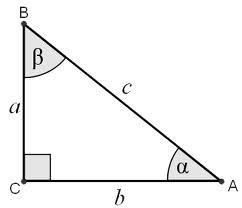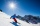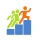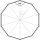# Right

Determine angles of the right triangle with the hypotenuse c and legs a, b, if:

$3a+4b=4.9c$

Correct result:

α =  64.6 °
β =  25.4 °
γ =  90 °

#### Solution:

$\ \\ 3a +4b = 4.9c \ \\ \ \\ c=1 \ \\ 3a +4b = 4.9 \ \\ a^2+b^2 =1 \ \\ \ \\ a=\dfrac{ 4.9 - 4 b}{ 3} \ \\ \ \\ \dfrac{ (4.9 - 4 b)^2}{ 3^2} + b^2 =1 \ \\ (4.9 - 4 b)^2 + 3^2 b^2 = 3^2 \ \\ \ \\ \ \\ 25b^2 -39.2b +15.01 =0 \ \\ \ \\ p=25; q=-39.2; r=15.01 \ \\ D = q^2 - 4pr = 39.2^2 - 4\cdot 25 \cdot 15.01 = 35.64 \ \\ D>0 \ \\ \ \\ b_{1,2} = \dfrac{ -q \pm \sqrt{ D } }{ 2p } = \dfrac{ 39.2 \pm \sqrt{ 35.64 } }{ 50 } \ \\ b_{1,2} = 0.784 \pm 0.119398492453 \ \\ b_{1} = 0.903398492453 \ \\ b_{2} = 0.664601507547 \ \\ \ \\ \text{ Factored form of the equation: } \ \\ 25 (b -0.903398492453) (b -0.664601507547) = 0 \ \\ \ \\ \ \\ \alpha = \arcsin b = 64.6 ^\circ$
$\beta =\mathrm{arccos}b=25.{4}^{\circ }$
$\gamma =9{0}^{\circ }$Our examples were largely sent or created by pupils and students themselves. Therefore, we would be pleased if you could send us any errors you found, spelling mistakes, or rephasing the example. Thank you!

Please write to us with your comment on the math problem or ask something. Thank you for helping each other - students, teachers, parents, and problem authors.Tips to related online calculators
Looking for help with calculating roots of a quadratic equation?
Pythagorean theorem is the base for the right triangle calculator.

#### You need to know the following knowledge to solve this word math problem:

We encourage you to watch this tutorial video on this math problem:

## Next similar math problems:

• SailboatThe 20 m long sailboat has an 8 m high mast in the middle of the deck. The top of the mast is fixed to the bow and stern with a steel cable. Determine how much cable is needed to secure the mast and what angle the cable will make with the ship's deck.
• Tetrahedral pyramid 8Let’s all side edges of the tetrahedral pyramid ABCDV be equally long and its base let’s be a rectangle. Determine its volume if you know the deviations A=40° B=70° of the planes of adjacent sidewalls and the plane of the base and the height h=16 of the p
• Black diamond runTaleah is skiing down a black diamond run. She begins skiing at the top of a ski trail whose elevation is about 8625 feet. The ski run ends toward the base of the mountain at 3800 feet. The horizontal distance between these two points is about 4775 feet.
• Traffic signThere is a traffic sign for climbing on the road with an angle of 7%. Calculate at what angle the road rises (falls).
• The basesThe bases of the isosceles trapezoid ABCD have lengths of 10 cm and 6 cm. Its arms form an angle α = 50˚ with a longer base. Calculate the circumference and content of the ABCD trapezoid.
• Powerplant chimneyFrom the window of the building at a height of 7.5 m, the top of the factory chimney can be seen at an altitude angle of 76° 30 ′. The base of the chimney can be seen from the same place at a depth angle of 5° 50 ′. How tall is the chimney?
• An observerAn observer standing west of the tower sees its top at an altitude angle of 45 degrees. After moving 50 meters to the south, he sees its top at an altitude angle of 30 degrees. How tall is the tower?
• The towerThe observer sees the base of the tower 96 meters high at a depth of 30 degrees and 10 minutes and the top of the tower at a depth of 20 degrees and 50 minutes. How high is the observer above the horizontal plane on which the tower stands?
• FighterA military fighter flies at an altitude of 10 km. From the ground position, it was aimed at an altitude angle of 23° and 12 seconds later at an altitude angle of 27°. Calculate the speed of the fighter in km/h.
• TV towerCalculate the height of the television tower if an observer standing 430 m from the base of the tower sees the peak at an altitude angle of 23°?The ladder touch on a wall at a height of 7.5 m. The angle of the inclination of the ladder is 76°. How far is the lower end of the ladder from the wall?From the observation tower at a height of 105 m above sea level, the ship is aimed at a depth angle of 1° 49´. How far is the ship from the base of the tower?From point A, the power line pole is seen at an angle of 18 degrees. From point B to which we get when going from point A 30m away from the column at an angle of 10 degrees. Find the height of the power pole.The rescue helicopter is above the landing site at a height of 180m. The site of the rescue operation can be seen from here at a depth angle of 52° 40 '. How far will the helicopter land from the rescue site?Find the height between the two floors if you know that the number of steps between the two floors is 18, the gradient is 30º and the length of the step is 28.6 cm. Report the result in centimeters to the nearest centimeter.Which of the following numbers most accurately area of a regular decagon with side s = 2 cm? (A) 9.51 cm2 (B) 20 cm2 (C) 30.78 cm2 (D) 31.84 cm2 (E) 32.90 cm2Calculate the volume (V) and the surface (S) of a regular quadrilateral prism whose height is 28.6 cm and the deviation of the body diagonal from the base plane is 50°.